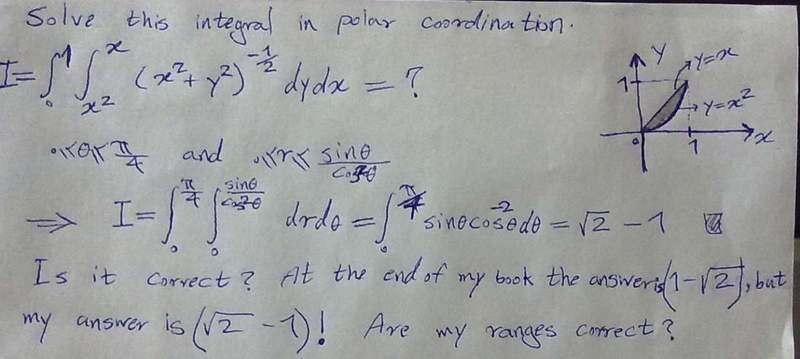# Double integral in polar coordination

## Homework Statement## The Attempt at a Solution

Please tell me if I am wrong. I suspect about the ranges. Are my range corrrect?

#### Attachments

Last edited:

Please tell me if I am wrong. I suspect about the ranges. Are my range corrrect?

If you have a double integral on the form I = $$\int \int_{R} f(x,y) dy dx$$
Then if you to integrate using polar coordinates then it changes to
I = $$\int \int_{R} f(x,y) dA$$ where

$$dA = r dr d\theta$$

trying adding this and see if you don't get the right result.

Dear Susanne217,

Thank you very much for your reply, but i have already added $$dA = r dr d\theta$$
to get the result. If you calculate it you will see that I had already added it! But my problem is the ranges. I suspect about the ranges.

The quantity you integrate (that is '1') is always positive.
An integral is basically a sum, so a sum of positive terms cannot turn into something negative.
The result of the book $$(1-\sqrt2 \cong -0.41)$$ is negative, so it is wrong.

$$=\int_0^{\pi/4} \int_{0}^{\frac{sen\theta}{cos^2\theta}} dr\: d\theta$$

$$=\int_0^{\pi/4} {\frac{sen\theta}{cos^2\theta}} \: d\theta$$

$$=\left[{\frac{1}{cos\theta}\right]_0^{\pi/4}$$

$$=\sqrt2-1$$

Last edited:
The quantity you integrate (that is '1') is always positive.
An integral is basically a sum, so a sum of positive terms cannot turn into something negative.
The result of the book $$(1-\sqrt2 \cong -0.41)$$ is negative, so it is wrong.

Thank you very much for the notification about "positive". But would you please tell me what your idea is about the ranges? especially about the ranges of "r". Are you sure that my ranges are correct?

Yes they are correct because in a polar-cartesian system:
the system
$$y = x tg\theta$$
$$y = x^2$$

gives $$x = tg\theta$$

$$r = \sqrt(x^2+y^2)= tg\theta \sqrt(tg^2\theta+1) = tg\theta / cos\theta = sen\theta / cos^2\theta$$

Dear Quinzio,

Thank you very much for your help.